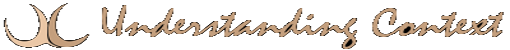# 26 Mar Bayes’ TheoremBayes’ Theorem is a mathematical equation for calculating evidence-based probability.  Reverend Thomas Bayes, University of Edinburgh (1701-1761) described how the probability (P) of a specific theoretical (T) outcome is affected by a new piece of evidence (E). The beauty of this theory and its use in conceptual networks is that it does not limit the number of constraints you can apply to solving a problem or obtaining an outcome. (There’s no surviving likeness of the man)

Mathematical Formulas from Wolfram

Better Explained

Bayesian Network

probability

inference

constraint

This site uses Akismet to reduce spam. Learn how your comment data is processed.